Courses

# NCERT Solutions(Part - 1) - Practical Geometry Class 6 Notes | EduRev

## Class 6 : NCERT Solutions(Part - 1) - Practical Geometry Class 6 Notes | EduRev

The document NCERT Solutions(Part - 1) - Practical Geometry Class 6 Notes | EduRev is a part of the Class 6 Course Mathematics (Maths) Class 6.
All you need of Class 6 at this link: Class 6

Exercise 14.1
Question 1: Draw a circle of radius 3.2 cm.
Steps of construction:
(a) Open the compass for the required radius of 3.2 cm.
(b) Make a point with a sharp pencil where we want the centre of circle to be.
(c) Name it O.
(d) Place the pointer of compasses on O.
(e) Turn the compasses slowly to draw the circle.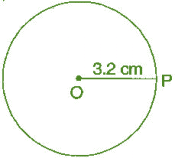Hence, it is the required circle.

Question 2: With the same centre O, draw two circles of radii 4 cm and 2.5 cm.
Steps of construction:
(a) Marks a point ‘O’ with a sharp pencil where we want the centre of the circle.
(b) Open the compasses 4 cm.
(c) Place the pointer of the compasses on O.
(d) Turn the compasses slowly to draw the circle.
(e) Again open the compasses 2.5 cm and place the pointer of the compasses on D.
(f) Turn the compasses slowly to draw the second circle.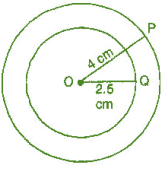Hence, it is the required figure.

Question 3: Draw a circle and any two of its diameters. If you join the ends of these diameters, what is the figure obtained? What figure is obtained if the diameters are perpendicular to each other? How do you check your answer?
(i) By joining the ends of two diameters, we get a rectangle. By measuring, we find AB = CD = 3 cm, BC = AD = 2 cm i.e., pairs of opposite sides are equal and also ∠A = ∠B = ∠C = ∠D = 90o  i.e., each angle is of 90o.
Hence, it is a rectangle.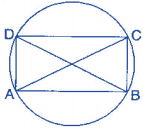(ii) If the diameters are perpendicular to each other, then by joining the ends of two diameters, we get a square. By measuring, we find that AB = BC = CD = DA = 2.5 cm, i.e., all four sides are equal. Also ∠A = ∠B = ∠C = ∠D = 90o, i.e., each angle is of 90o.
Hence, it is a square.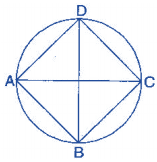Question 4: Draw any circle and mark points A, B and C such that:
(a) A is on the circle.
(b) B is in the interior of the circle.
(c) C is in the exterior of the circle.
(i) Mark a point ‘O’ with sharp pencil where we want centre of the circle.
(ii) Place the pointer of the compasses at ‘O’. Then move the compasses slowly to draw a circle.
(a) Point A is on the circle.
(b) Point B is in interior of the circle.
(c) Point C is in the exterior of the circle.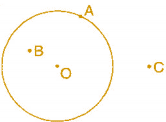Question 5: Let A, B be the centres of two circles of equal radii; draw them so that each one of them passes through the centre of the other. Let them intersect at C and D.
Examine whether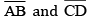are at right angles.
Draw two circles of equal radii taking A and B as their centre such that one of them passes through the centre of the other. They intersect at C and D. Join AB and CD.
Yes, AB and CD intersect at right angle as ∠COB is 90o .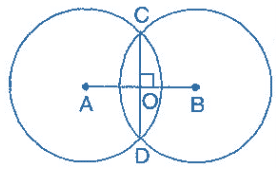Exercise 14.2
Question 1: Draw a line segment of length 7.3 cm, using a ruler.
Steps of construction: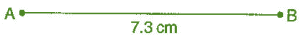(i) Place the zero mark of the ruler at a point A.
(ii) Mark a point B at a distance of 7.3 cm from A.
(iii) Join AB.
Hence,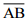is the required line segment of length 7.3 cm.

Question 2: Construct a line segment of length 5.6 cm using ruler and compasses.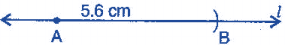(i) Draw a line 'l'. l Mark a point A on this line.
(ii) Place the compasses pointer on zero mark of the ruler. Open it to place the pencil point up to 5.6 cm mark.
(iii) Without changing the opening of the compasses. Place the pointer on A and cut an arc 'l'  at B.is the required line segment of length 5.6 cm.

Question 3:
Constructof length 7.8 cm. From this, cut off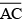of length 4.7 cm. Measure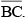Steps of construction: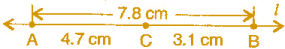(i) Place the zero mark of the ruler at A.
(ii) Mark a point B at a distance 7.8 cm from A.
(iii) Again, mark a point C at a distance 4.7 from A.
Hence, by measuring, we find that BC = 3.1 cm.

Question 4: Givenof length 3.9 cm, construct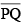such that the length ofis twice that of. Verify by measurement.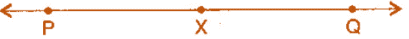(Hint: construct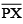such that length of= length of; then cut off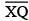such thatalso has the length of.)
Steps of construction: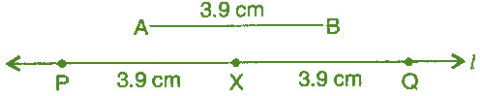(i) Draw a line 'l'.
(ii) Construct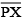such that length of= length of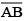(iii) Then cut of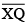such thatalso has the length of.
(iv) Thus the length ofand the length of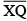added together make twice the length of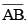Verification: Hence, by measurement we find that PQ = 7.8 cm
= 3.9 cm + 3.9 cm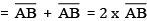Question 5: Givenof length 7.3 cm and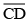of length 3.4 cm, construct a line segment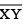such that the length ofis equal to the difference between the lengths ofVerify by measurement.
Steps of construction:
(i) Draw a line 'l' and take a point X on it.
(ii) Construct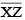such that length= length of= 7.3 cm
(iii) Then cut off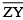= length of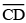= 3.4 cm
(iv) Thus the length of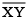= length of– length of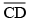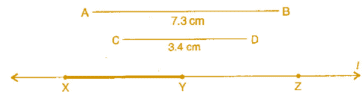Verification:
Hence, by measurement we find that length of= 3.9 cm
= 73. cm – 3.4 cm
=-Exercise 14.3
Question 1:
Draw any line segment. Without measuring, construct a copy of.
Steps of construction: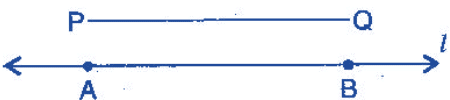(i) Givenwhose length is not known.
(ii) Fix the compasses pointer on P and the pencil end on Q. The opening of the instrument now gives the length of.
(iii) Draw any line 'l'. Choose a point A on 'l'. Without changing the compasses setting, place the pointer on A.
(iv) Draw an arc that cuts 'l' at a point, say B.
Hence,is the copy of.

Question 2:
Given some line segment, whose length you do not know, constructsuch that the length ofis twice that of.
Steps of construction: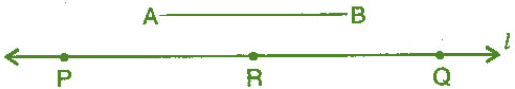(i) Givenwhose length is not known.
(ii) Fix the compasses pointer on A and the pencil end on B. The opening of the instrument now gives the length of.
(iii) Draw any line 'l'. Choose a point P on 'l'. Without changing the compasses setting, place the pointer on Q.
(iv) Draw an arc that cuts 'l' at a point R.
(v) Now place the pointer on R and without changing the compasses setting, draw another arc that cuts 'l' at a point Q.
Hence,is the required line segment whose length is twice that of AB.

Offer running on EduRev: Apply code STAYHOME200 to get INR 200 off on our premium plan EduRev Infinity!

## Mathematics (Maths) Class 6

185 videos|229 docs|43 tests

,

,

,

,

,

,

,

,

,

,

,

,

,

,

,

,

,

,

,

,

,

;